# NPV Formula

Difference between the current value of future cash flows and the cost of launching the project.

The net present value of an investment is the difference between the current value of future cash flows and the cost of launching the project. Whenever the net present value exceeds the price, the project has created value for the company and its shareholders.Usually, NPV is the difference between the present value of the future cash flows of the investment and the initial cost of the investment.

Net present value is based on the time value of money, the idea that a dollar today is worth more than a dollar due to factors like inflation, opportunity cost, etc. To arrive at NPV, all future cash flows are reduced in value at a "discount rate" to arrive at the "present value" of the investment.

Using net present value to evaluate an investment is a critical objective in capital budgeting. Everything that financial managers decide to do (e.g., taking on investments or launching projects) should be aimed at creating value for the company and its shareholders.

The basic idea of creating value revolves around the concept that we should identify an investment worth more than it initially cost us to acquire. Once the market value exceeds the initial cost, then it is said that the project has created value for the investor.

The net present value of a project quantifies the value created in a company in dollar terms:

• An investment with a positive NPV indicates that it will create value, so investing is advisable.
• An investment with a negative NPV indicates reduced value, so it is advisable not to invest.
• An investment with an NPV equal to 0 indicates that it has neither created nor reduced the value of the business, so managers are indifferent to investing.

Therefore, the net present value rule states, "When the net present value of an investment is positive, it should be accepted; if it is negative, it should be rejected." NPV is one of the most famous and reliable methods of evaluating the return on investment.

## Understanding net present value

A business will elect to invest if an opportunity generates value greater than its initial cost. The investment is said to have created value when a project's cost is less than its market worth. However, determining the market value calls for accurate estimation techniques.For example, say you bought an empty studio for $200,000. Since you are a great interior designer, you furnished the condo's interior neatly for$40,000. In addition, you installed the necessary furniture and appliances required for someone to live inside it.

The studio was bought for $200,000, and the total renovations cost$40,000. Therefore, the total initial investment amounts to $240,000. However, being an expert broker, you were able to sell the apartment in the market for$300,000, $60,000 more than the initial investment. The market value of the studio ($300,000) exceeds its cost ($240,000) by$60,000. That difference is the value created due to strong management: buying the condo, getting materials to furnish it, and making it livable. This management has created $60,000 in value. This is undoubtedly a significant investment to undertake. However, the difficult part is estimating that the renovated apartment would sell for$300,000. Every business and company try to evaluate whether an investment is worth it based on the assessed value it creates.

The net profit of this investment is the difference between the market value of the investment and the cost of acquiring it. In our previous case, net profit is positive since the asset's market value exceeds its cost by $60,000. What if we can't observe the market value of the investment? What if profits will only be fully realized far into the future? How will we account for the time value of money? We try to use indirect market information, like the estimated cash inflows of the investment, to calculate the NPV of a project. This is why calculating NPV requires a discounted cash flow analysis. ## Method for calculating the net present value In the previous example, we somehow knew how much our$240,000 investment would be worth at some point in the future. But unfortunately, all managers and investors are subject to imperfect market information.That is to say, instead of knowing how much it would sell for, financial decision-makers would estimate the cash flows that the project will bring into the business and then try to decide whether or not it's worth undertaking that investment.

Instead of comparing the market value of an investment with its initial cost, this method requires finding the present value of the cash inflows that the investment will bring into the company. Then, this current value will be compared with the project's cost.

• The investment should be taken if the cash flow's present value exceeds the project's cost.
• If the present value of the cash flows is less than the cost of launching the project, then the investment should not be taken because the value of the business will be reduced.
• If the present value of the cash flows is equal to the cost of launching the project, then managers are indifferent to taking the investment, as no deal will be created.

For example, a manager is considering launching a project to create value for the corporation's shareholders. However, the project is unique and has not been done before in the industry, so the manager doesn't know the investment's market value.Instead, the manager can only estimate how much cash the project will bring into the company over the next five years. After some calculations, the executive forecasted the present value of the cash flows would be worth $100,000. To launch the project, the company would have to spend$110,000. The cost of undertaking the investment exceeds the present value of the project's cash inflows by $10,000. Therefore, the manager refrains from launching the project because it will reduce the company's value. ## The NPV formula As previously mentioned, calculating the net present value of investment requires comparing the current value of the cash flows that the project brings in with the initial cost of launching the project. Therefore, the first step in calculating it is knowing how to calculate the present value.For context, the present value is the current value of an amount of money that will be received in the future (i.e., a series of future cash inflows). So, for example, if you were to receive$1,000 one year from now, the current value is how much that $1,000 promise is worth today. We perform a discounted cash flow (DCF) analysis to do this. We need a discount rate to be able to forgive future sums of money to the current period. In a DCF analysis, the discount rate is the interest rate used to calculate the present value of future cash flows. To calculate the PV of future cash flows, we use the following formula: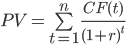• CF(t) is the net cash flow (cash inflow - cash outflow) that the investment gives at year t • r is the discount rate Despite the daunting appearance of this formula, it is merely determining the present value of each cash flow individually. The total present value of the investment is then calculated by summing up all the current deals to see whether the project is profitable.For example, suppose an investment has the following cash inflows: 1.$5,000 at the end of the first year
2. $6,000 at the end of the second year 3.$10,000 at the end of the third year

With a discount rate of 7%, the present value of the three future cash flows will be: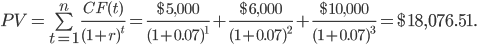Therefore, the future cash flows are worth $18,076.51 today. In other words, we can say that the market value of the investment is$18,076.51 today. If we want to find the investment's net present value, we compare this PV to the initial cost.

Therefore, the formula for finding the net present value of an investment is: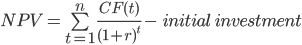## Examples

Let's say a manufacturing business is thinking about funding an investment. The project's eventual market value is uncertain at this time. However, with accuracy, the firm can predict the cash inflows this investment will bring.1. At the end of the first year, the project will bring a cash revenue of $50,000 2. At the end of the second year, the project will get a cash revenue of$76,000
3. At the end of the third year, the project will get a cash revenue of $85,000 4. The project costs$150,000 to launch
5. The company uses a discount rate of 10%

By using the formula: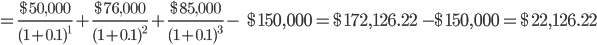We now know that, at year 0, the project has a market value of $172,126.22. Moreover, the project initially cost the company$150,000. With these forecasts, suffice to say, the investment is worth it because it creates more money than the firm initially dedicated to it.

This means that the project has a positive net present value. We reached this conclusion by subtracting the initial cost of the investment from its current market value, and the resulting number was positive.

Since it is a positive number, the company should accept the project and invest. This is the main rule of thumb for a positive NPV. On the other hand, if the NPV were negative, the company would have rejected the project and not invested.

Let's go on with another, more complicated example. Suppose an auto company is considering establishing an auto repair booth. It knows how much it will cost to launch the project and how much cash revenue it will provide over the next six years.It believes the repair booth will provide $50,000 in revenue annually. It also estimates that the booth's annual cost is$15,000. When the company closes this side business after six years, the salvage value of the equipment left will be $10,000. What decision should the auto manufacturer make regarding launching the project, provided they use a discount rate of 12% for such investments? In other words, what is the net present value of this investment? The net cash inflow that this side business will bring in every six years is$50,000 - $15,000 =$35,000, except for the sixth year, where the additional $10,000 of salvage value should be added. Therefore, the net cash inflow at the end of year 6 is$45,000.

Therefore, we should find the present value of that series of cash flows and compare it to the initial cost of launching the repair booth. The first five cash flows represent an annuity in equal periodic payments.

Therefore, we can find the present value of the first five cash flows using the annuity formula, and then we manually see the present value of the sixth cash flow.

Finally, we add both figures together to find the project's current value and compare it with the cost.

The formula for the present value of an annuity is as follows: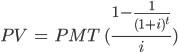• PMT is the annual cash flow of the investment
• i is the discount rate
• t is the length of the annuity in years

Therefore, the present value of the first five cash flows is: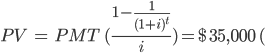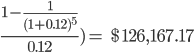To find the present value of this investment, we also discount the sixth cash flow and add it to the PV of the annuity we just saw. Afterward, we subtract the initial cost of the investment from the present value to find the NPV.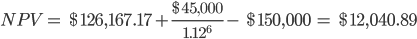Therefore, this project has created $12,040.89 in value. If the company had 10,000 shares, for instance, this project would impact the price of the stock by$12,040.89 / 10,000 = $1.2. With this estimated net present value, we can expect a$1.2 increase in share price.### Everything You Need To Master DCF Modeling

To Help You Thrive in the Most Prestigious Jobs on Wall Street.

Researched and authored by Vatche Tchelderian | LinkedIn